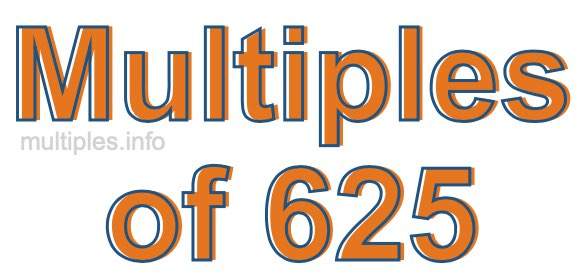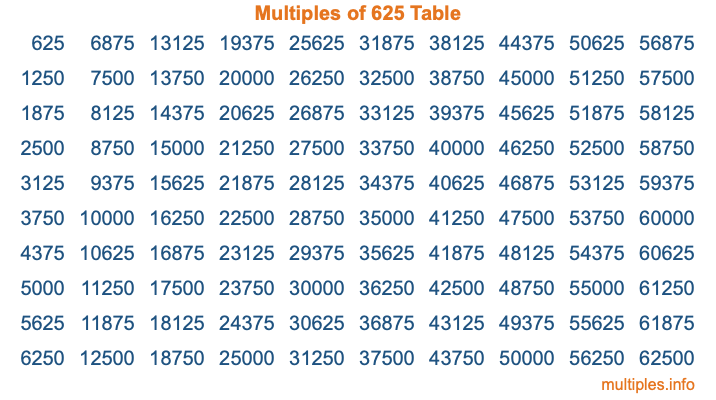Multiples of 625Welcome to the Multiples of 625 page. Here we will first teach you everything you will ever need to know about the multiples of 625, and then give you a study guide summary of everything we taught you to make sure you remember it all. Use this page to look up facts and learn information about the multiples of 625. This page will make you a multiples of six hundred twenty-five expert!

Definition of Multiples of 625
Multiples of 625 are all the numbers that when divided by 625 equal an integer. Each of the multiples of 625 are called a multiple. A multiple of 625 is created by multiplying 625 by an integer.

Therefore, to create a list of multiples of 625, you start with 1 multiplied by 625, then 2 multiplied by 625, then 3 multiplied by 625, and so on for as long as you want. Thus, the list of the first five multiples of 625 is 625, 1250, 1875, 2500, and 3125. To see a larger list of multiples of 625, see the printable image of Multiples of 625 further down on this page. We also have a category where you can choose any nth multiple of 625.

Multiples of 625 Checker
The Multiples of 625 Checker below checks to see if any number of your choice is a multiple of 625. In other words, it checks to see if there is any number (integer) that when multiplied by 625 will equal your number. To do that, we divide your number by 625. If the the quotient is an integer, then your number is a multiple of 625.

Is  a multiple of 625?

Least Common Multiple of 625 and ...
A Least Common Multiple (LCM) is the lowest multiple that two or more numbers have in common. This is also called the smallest common multiple or lowest common multiple and is useful to know when you are adding our subtracting fractions. Enter one or more numbers below (625 is already entered) to find the LCM.

Check out our LCM Calculator if you need more details about the Least Common Multiple or if you need the LCM for different numbers for adding and subtraction fractions.

nth Multiple of 625
As we stated above, 625 is the first multiple of 625, 1250 is the second multiple of 625, 1875 is the third multiple of 625, and so on. Enter a number below to find the nth multiple of 625.

th multiple of 625

Multiples of 625 vs Factors of 625
625 is a multiple of 625 and a factor of 625, but that is where the similarities end. All postive multiples of 625 are 625 or greater than 625. All positive factors of 625 are 625 or less than 625.

Below is the beginning list of multiples of 625 and the factors of 625 so you can compare:

Multiples of 625: 625, 1250, 1875, 2500, 3125, etc.

Factors of 625: 1, 5, 25, 125, 625

As you can see, the multiples of 625 are all the numbers that you can divide by 625 to get a whole number. The factors of 625, on the other hand, are all the whole numbers that you can multiply by another whole number to get 625.

It's also interesting to note that if a number (x) is a factor of 625, then 625 will also be a multiple of that number (x).

Multiples of 625 vs Divisors of 625
The divisors of 625 are all the integers that 625 can be divided by evenly. Below is a list of the divisors of 625.

Divisors of 625: 1, 5, 25, 125, 625

The interesting thing to note here is that if you take any multiple of 625 and divide it by a divisor of 625, you will see that the quotient is an integer.

Multiples of 625 Table
Below is an image of the first 100 multiples of 625 in a table. The table is in chronological order, column by column. The first column has the first ten multiples of 625, the second column has the next ten multiples of 625, and so on.The Multiples of 625 Table is also referred to as the 625 Times Table or Times Table of 625. You are welcome to print out our table for your studies.

Negative Multiples of 625
Although not often discussed or needed in math, it is worth mentioning that you can make a list of negative multiples of 625 by multiplying 625 by -1, then by -2, then by -3, and so on, to get the following list of negative multiples of 625:

-625, -1250, -1875, -2500, -3125, etc.

Multiples of 625 Summary
Below is a summary of important Multiples of 625 facts that we have discussed on this page. To retain the knowledge on this page, we recommend that you read through the summary and explain to yourself or a study partner why they hold true.

There are an infinite number of multiples of 625.

A multiple of 625 divided by 625 will equal a whole number.

625 divided by a factor of 625 equals a divisor of 625.

The nth multiple of 625 is n times 625.

The largest factor of 625 is equal to the first positive multiple of 625.

625 is a multiple of every factor of 625.

625 is a multiple of 625.

A multiple of 625 divided by a divisor of 625 equals an integer.

625 divided by a divisor of 625 equals a factor of 625.

Any integer times 625 will equal a multiple of 625.

Multiples of a Number
Here you can get the multiples of another number, all with the same attention to detail as we did for multiples of 625 on this page.

Multiples of
Multiples of 626
Did you find our page about multiples of six hundred twenty-five educational? Do you want more knowledge? Check out the multiples of the next number on our list!